# Differential Expression Inference

Once the reads have been mapped and counted, one can assess the differential expression of genes between different conditions.

During this lesson, you will learn to :

• describe the different steps of data normalization and modelling commonly used for RNA-seq data.
• detect significantly differentially-expressed genes using either edgeR or DESeq2.
• perform downstream analysis on gene sets, such as annotation (e.g. GO terms or Reactome pathways) over-representation.

## Material

Rstudio website

Note

RStudio is set to be rebranded as Posit after October 2022.

## Connexion to the Rstudio server

Note

This step is intended only for users who attend the course with a teacher. Otherwise you will have to rely on your own installation of Rstudio.

The analysis of the read count data will be done on an RStudio instance, using the R language and some relevant Bioconductor libraries.

As you start your session on the RStudio server, please make sure that you know where your data is situated with respect to your working directory (use `getwd()` and `setwd()` to respectively : know what your working is, and change it as necessary).

## Differential Expression Inference

Use either edgeR or DESeq2 to conduct a differential expression analysis on the Ruhland2016 and/or Liu2015 dataset.

You can find the expression matrices on the server at: `/shared/data/Solutions/Ruhland2016/countFiles/featureCounts_Ruhland2016.counts.txt` and `/shared/data/Solutions/Liu2015/countFiles/featureCounts_Liu2015.counts.txt`

Note

• Generally, users find the syntax and workflow of DESeq2 easier for getting started.
• If you have the time, conduct a differential expression analysis using both DESeq2 and edgeR.
• Follow the vignettes/user’s guide! They are the most up-to-date and generally contain everything a newcomer might need, including worked-out examples.

### DESeq2

``````# setup
library(DESeq2)
library(ggplot2)

# setting up row names as ensembl gene ids
row.names(raw_counts) = raw_counts\$Geneid

## looking at the beginning of that table
raw_counts[1:5,1:5]

# removing these first columns to keep only the sample counts
raw_counts = raw_counts[ ,  -1:-6  ]

# changing column names
names( raw_counts) = gsub('_.*', '', gsub('.*.SRR[0-9]{7}_', '', names(raw_counts) ) )

# some checking of what we just read
colSums(raw_counts) # total number of counted reads per sample
``````
preprocessing
``````## telling DESeq2 what the experimental design was
# note: by default, the 1st level is considered to be the reference/control/WT/...
treatment <- factor( c(rep("EtOH",3), rep("TAM",3)), levels=c("EtOH", "TAM") )
colData <- data.frame(treatment, row.names = colnames(raw_counts))
colData

## creating the DESeq data object & positing the model
dds <- DESeqDataSetFromMatrix(
countData = raw_counts, colData = colData,
design = ~ treatment)
dim(dds)

## filter low count genes. Here, only keep genes with at least 2 samples where there are at least 5 reads.
idx <- rowSums(counts(dds, normalized=FALSE) >= 5) >= 2
dds.f <- dds[idx, ]
dim(dds.f)

# we go from 55414 to 19378 genes
``````

Around 19k genes pass our minimum expression threshold, quite typical for a bulk Mouse RNA-seq experiment.

estimate dispersion / model fitting
``````# we perform the estimation of dispersions
dds.f <- DESeq(dds.f)

# we plot the estimate of the dispersions
# * black dot : raw
# * red dot : local trend
# * blue : corrected
plotDispEsts(dds.f)

# extracting results for the treatment versus control contrast
res <- results(dds.f)
``````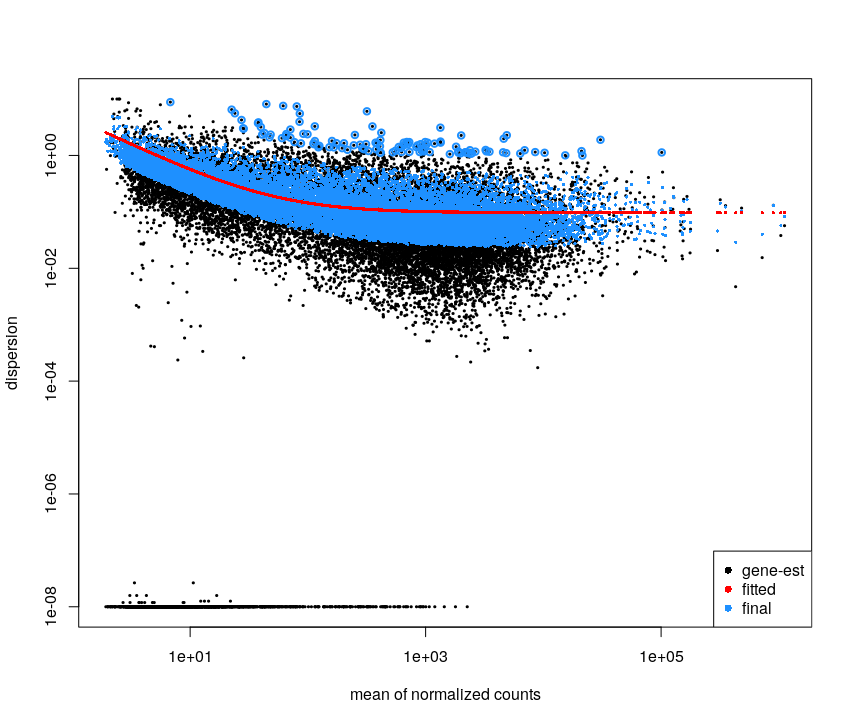This plot is not easy to interpret. It represents the amount of dispersion at different levels of expression. It is directly linked to our ability to detect differential expression.

Here it looks about normal compared to typical bulk RNA-seq experiments : the dispersion is comparatively larger for lowly expressed genes.

looking at the results
``````# adds estimate of the LFC the results table.
# This shrunk logFC estimate is more robust than the raw value

head(coef(dds.f)) # the second column corresponds to the difference between the 2 conditions
res.lfc <- lfcShrink(dds.f, coef=2, res=res)

#plotting to see the difference.
par(mfrow=c(2,1))
DESeq2::plotMA(res)
DESeq2::plotMA(res.lfc)
# -> with shrinkage, the significativeness and logFC are more consistent
par(mfrow=c(1,1))
``````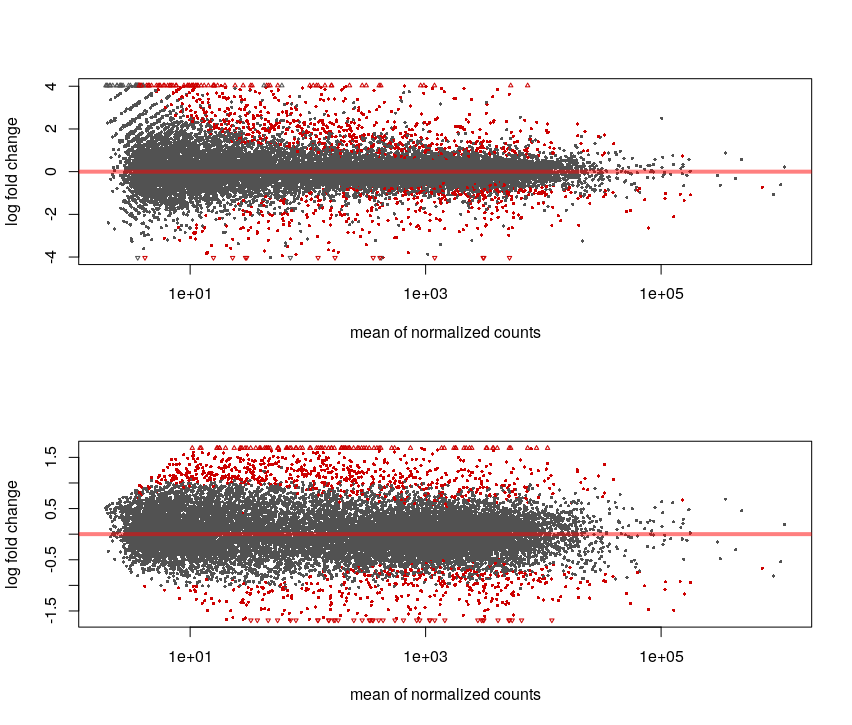Without the shrinkage, we can see that for low counts we can see a high log-fold change but non significant (ie. we see a large difference but with variance is also so high that this observation may be due to chance only).

The shrinkage corrects this and the relationship between logFC and significance is smoother.

``````# we apply the variance stabilising transformation to make the read counts comparable across libraries
# (nb : this is not needed for DESeq DE analysis, but rather for visualisations that compare expression across samples, such as PCA. This replaces normal PCA scaling)
vst.dds.f <- vst(dds.f, blind = FALSE)
vst.dds.f.counts <- assay(vst.dds.f)

plotPCA(vst.dds.f, intgroup = c("treatment"))
``````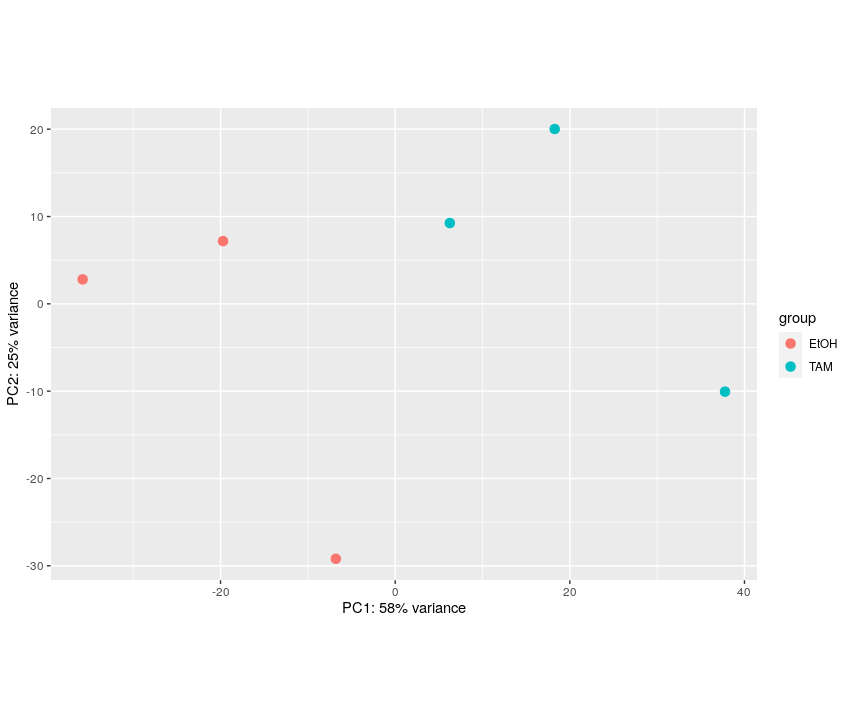The first axis (58% of the variance) seems linked to the grouping of interest.

``````## ggplot2-based volcano plot
library(ggplot2)

FDRthreshold = 0.01
logFCthreshold = 1.0
# add a column of NAs
res.lfc\$diffexpressed <- "NO"
# if log2Foldchange > 1 and pvalue < 0.01, set as "UP"
res.lfc\$diffexpressed[res.lfc\$log2FoldChange > logFCthreshold & res.lfc\$padj < FDRthreshold] <- "UP"
# if log2Foldchange < 1 and pvalue < 0.01, set as "DOWN"
res.lfc\$diffexpressed[res.lfc\$log2FoldChange < -logFCthreshold & res.lfc\$padj < FDRthreshold] <- "DOWN"

ggplot( data = data.frame( res.lfc ) , aes( x=log2FoldChange , y = -log10(padj) , col =diffexpressed ) ) +
geom_point() +
geom_vline(xintercept=c(-logFCthreshold, logFCthreshold), col="red") +
geom_hline(yintercept=-log10(FDRthreshold), col="red") +
scale_color_manual(values=c("blue", "grey", "red"))

table(res.lfc\$diffexpressed)
``````
`````` DOWN    NO    UP
131 19002   245
``````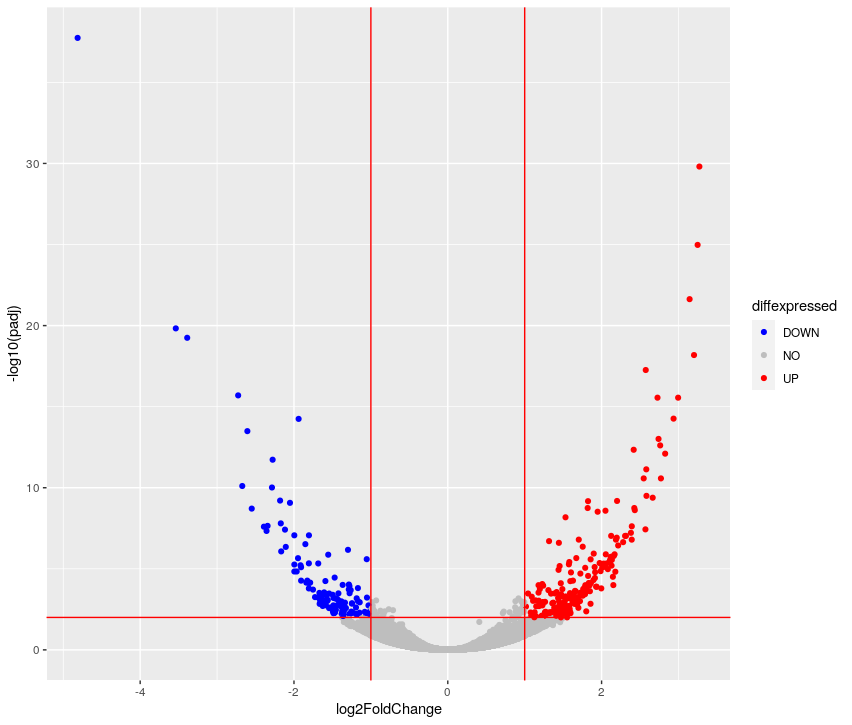``````library(pheatmap)
topVarGenes <- head(order(rowVars(vst.dds.f.counts), decreasing = TRUE), 20)
mat  <- vst.dds.f.counts[ topVarGenes, ] #scaled counts of the top genes
mat  <- mat - rowMeans(mat)  # centering
pheatmap(mat)
``````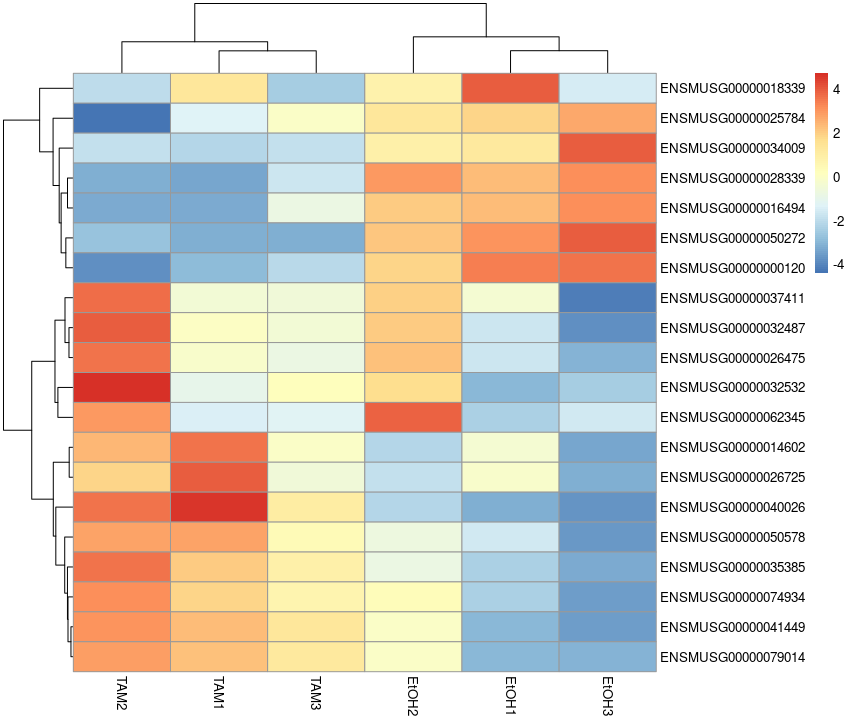``````# saving results to file
# note: a CSV file can be imported into Excel
write.csv( res ,'Ruhland2016.DESeq2.results.csv' )
``````

### EdgeR

``````library(edgeR)
library(ggplot2)

# setting up row names as ensembl gene ids
row.names(raw_counts) = raw_counts\$Geneid

# removing these first columns to keep only the sample counts
raw_counts = raw_counts[ ,  -1:-6  ]

# changing column names
names( raw_counts) = gsub('_.*', '', gsub('.*.SRR[0-9]{7}_', '', names(raw_counts) ) )

# some checking of what we just read
colSums(raw_counts) # total number of counted reads per sample
``````
edgeR object preprocessing
``````# setting up the experimental design AND the model
#  -> the first 3 samples form a group, the 3 remaining are the other group
treatment <-  c(rep("EtOH",3), rep("TAM",3))
dge.f.design <- model.matrix(~ treatment)

# creating the edgeR DGE object
dge.all <- DGEList(counts = raw_counts , group = treatment)

# filtering by expression level. See ?filterByExpr for details
keep <- filterByExpr(dge.all)
dge.f <- dge.all[keep, keep.lib.sizes=FALSE]
table( keep )
``````
``````keep
FALSE  TRUE
39702 15712
``````

Around 16k genes are sufficiently expressed to be retained.

``````#normalization
dge.f <- calcNormFactors(dge.f)
dge.f\$samples
``````

Each sample has been associated with a normalization factor.

edgeR model fitting

``````# estimate of the dispersion
dge.f <- estimateDisp(dge.f,dge.f.design , robust = T)
plotBCV(dge.f)
``````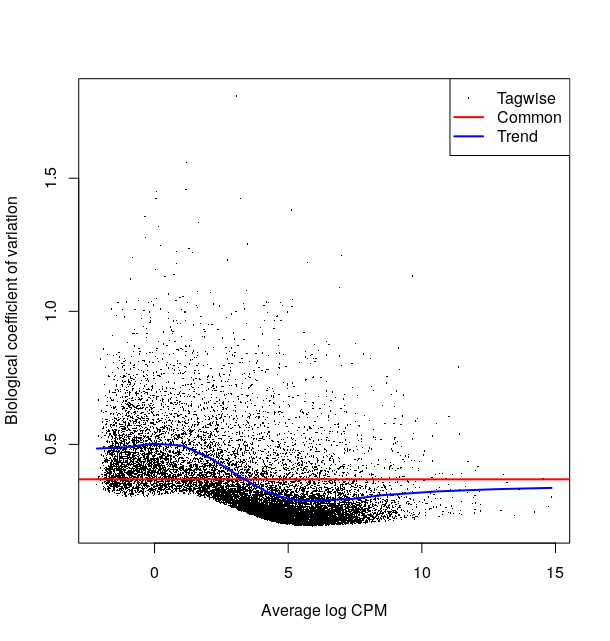This plot is not easy to interpret. It represents the amount of biological variation at different levels of expression. It is directly linked to our ability to detect differential expression.

Here it looks about normal compared to other bulk RNA-seq experiments : the variation is comparatively larger for lowly expressed genes.

``````# testing for differential expression.
# This method is recommended when you only have 2 groups to compare
dge.f.et <- exactTest(dge.f)
topTags(dge.f.et) # printing the genes where the p-value of differential expression if the lowest
``````
``````Comparison of groups:  TAM-EtOH
logFC   logCPM       PValue          FDR
ENSMUSG00000050272 -8.522762 4.988067 2.554513e-28 3.851950e-24
ENSMUSG00000075014  3.890079 5.175181 2.036909e-25 1.535728e-21
ENSMUSG00000009185  3.837786 6.742422 1.553964e-22 7.810743e-19
ENSMUSG00000075015  3.778523 3.274463 2.106799e-22 7.942107e-19
ENSMUSG00000028339 -5.692069 6.372980 4.593720e-16 1.385374e-12
ENSMUSG00000040111 -2.141221 6.771538 4.954522e-15 1.245154e-11
ENSMUSG00000041695  4.123972 1.668247 6.057909e-15 1.304960e-11
ENSMUSG00000072941  3.609170 7.080257 1.807618e-14 3.407135e-11
ENSMUSG00000000120 -6.340146 6.351489 2.507019e-14 4.200371e-11
ENSMUSG00000034981  3.727969 5.244841 3.934957e-14 5.933521e-11
``````
``````# see how many genes are DE
summary(decideTests(dge.f.et , p.value = 0.01)) # let's use 0.01 as a threshold
``````
``````         TAM-EtOH
Down     109
NotSig 15393
Up       210
``````

The comparison is TAM-EtOH, so “Up”, corresponds to a higher in group TAM compared to group EtOH.

edgeR looking at differentially-expressed genes
``````## plot all the logFCs versus average count size. Significantly DE genes are  colored
par(mfrow=c(1,1))
plotMD(dge.f.et)
# lines at a log2FC of 1/-1, corresponding to a shift in expression of x2
abline(h=c(-1,1), col="blue")
``````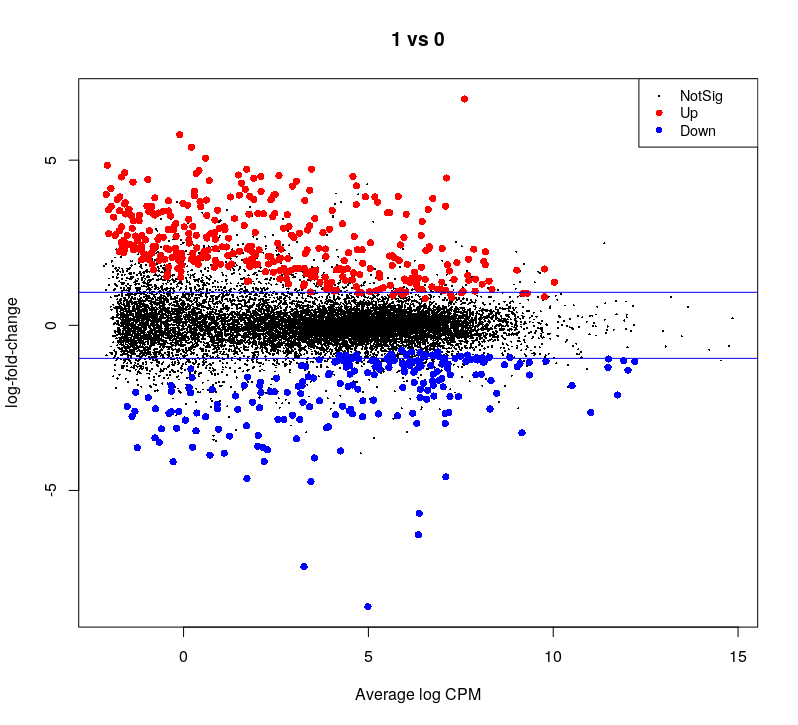``````## Volcano plot
allGenes = topTags(dge.f.et , n = nrow(dge.f.et\$table) )\$table

FDRthreshold = 0.01
logFCthreshold = 1.0
# add a column of NAs
allGenes\$diffexpressed <- "NO"
# if log2Foldchange > 1 and pvalue < 0.01, set as "UP"
allGenes\$diffexpressed[allGenes\$logFC > logFCthreshold & allGenes\$FDR < FDRthreshold] <- "UP"
# if log2Foldchange < 1 and pvalue < 0.01, set as "DOWN"
allGenes\$diffexpressed[allGenes\$logFC < -logFCthreshold & allGenes\$FDR < FDRthreshold] <- "DOWN"

ggplot( data = allGenes , aes( x=logFC , y = -log10(FDR) , col =diffexpressed ) ) +
geom_point() +
geom_vline(xintercept=c(-logFCthreshold, logFCthreshold), col="red") +
geom_hline(yintercept=-log10(FDRthreshold), col="red") +
scale_color_manual(values=c("blue", "grey", "red"))
``````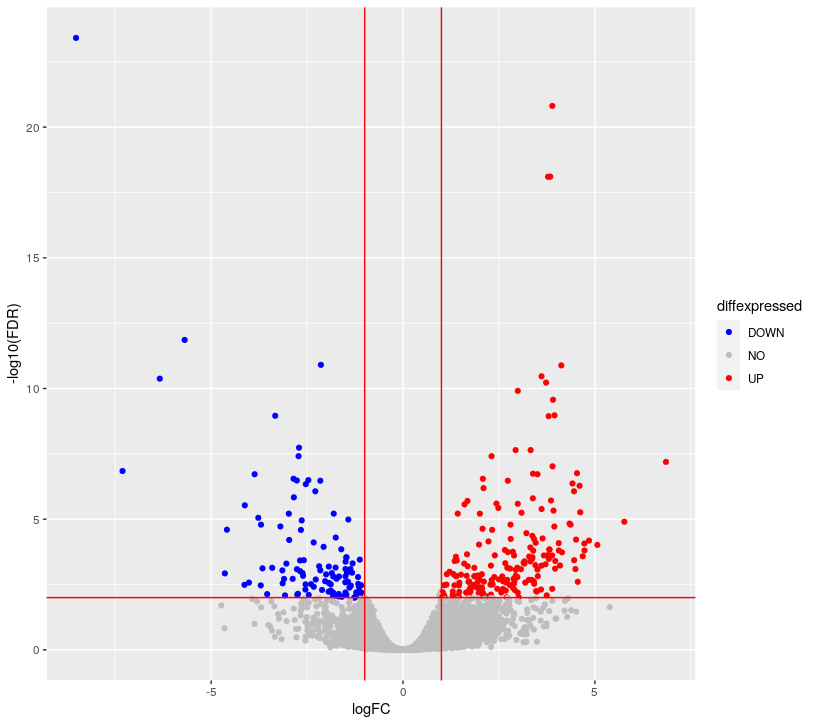``````## writing the table of results
write.csv( allGenes , 'Ruhland2016.edgeR.results.csv')
``````
edgeR extra stuff
``````# how to extract log CPM
logcpm <- cpm(dge.f, prior.count=2, log=TRUE)
``````
``````# there is another fitting method reliying on quasi-likelihood, which is useful when the model is more complex (ie. more than 1 factor with 2 levels)
dge.f.QLfit <- glmQLFit(dge.f, dge.f.design)
dge.f.qlt <- glmQLFTest(dge.f.QLfit, coef=2)

# you can see the results are relatively different. The order of genes changes a bit, and the p-values are more profoundly affected
topTags(dge.f.et)
topTags(dge.f.qlt)

## let's see how much the two methods agree:
par(mfrow=c(1,2))
plot( dge.f.et\$table\$logFC ,
dge.f.qlt\$table\$logFC,
xlab = 'exact test logFC',
ylab = 'quasi-likelihood test logFC')

print( paste('logFC pearson correlation coefficient :' ,
cor(dge.f.et\$table\$logFC ,dge.f.qlt\$table\$logFC) ) )

plot( log10(dge.f.et\$table\$PValue ),
log10(dge.f.qlt\$table\$PValue) ,
xlab = 'exact test p-values (log10)',
ylab = 'quasi-likelihood test p-values (log10)')

print( paste( "P-values spearman correlation coefficient",
cor( log10(dge.f.et\$table\$PValue ), log10(dge.f.qlt\$table\$PValue) , method = 'spearman' )))
``````
``````"logFC pearson correlation coefficient : 0.999997655536736"
"P-values spearman correlation coefficient 0.993238670517236"
``````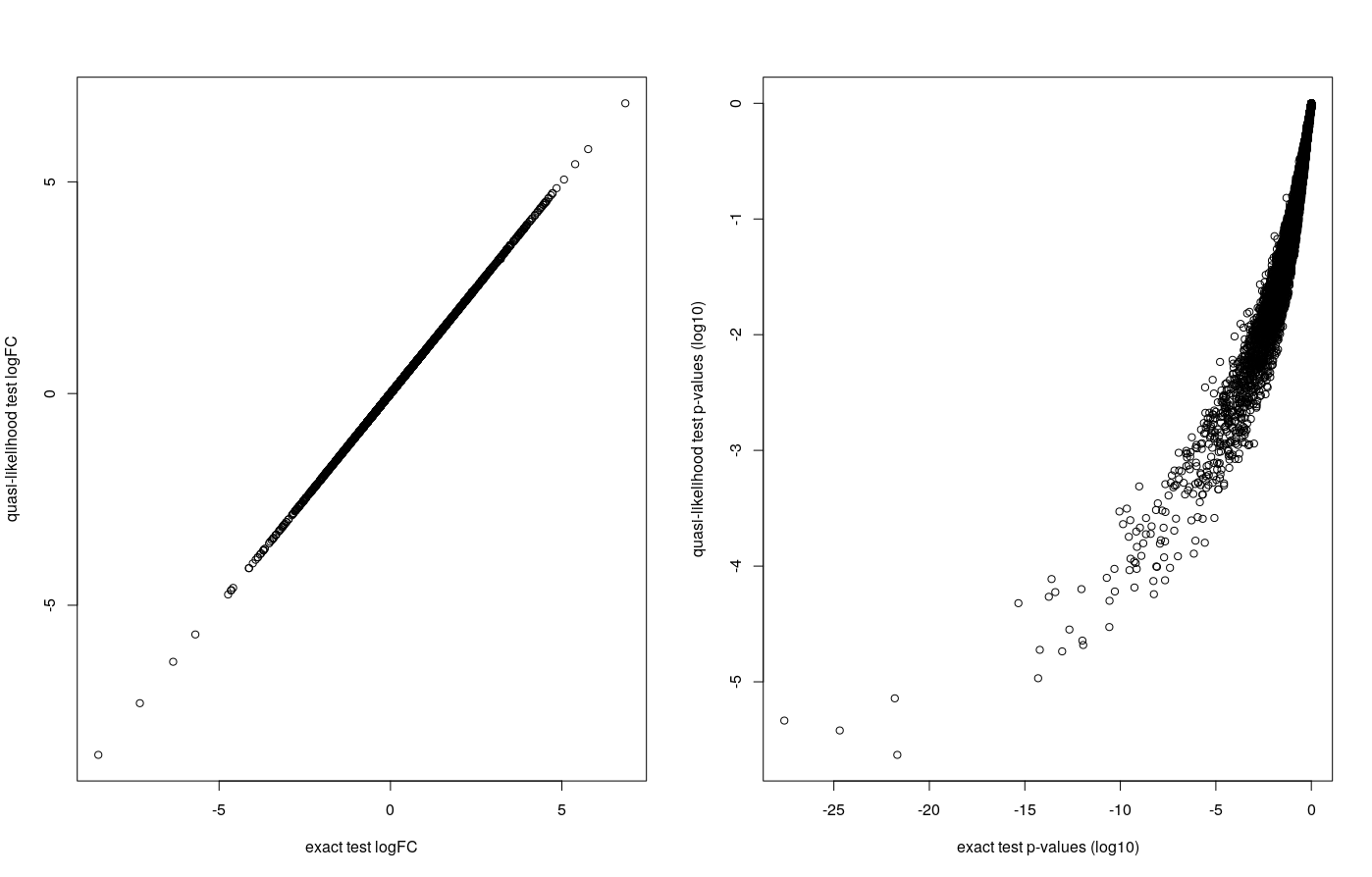The logFC are highly correlated. FDRs show less correlation but their rank are higly correlated : they come in a very similar order.

## Downstream analysis : over-representation analysis

Having lists of differentially-expressed genes is quite interesting in itself, however when there are many DE genes, it can be interesting to map these results onto curated sets of genes associated with known biological functions.

Here, we propose to use clusterProfiler, which regroups several enrichment detection algorithms onto several databases.

We recommend you get inspiration from their very nice vignette/e-book to perform your own analyses.

The proposed correction will concern the results obtained with DESeq2 on the Ruhland2016 dataset.

analysis with clusterProfiler

We begin by reading the results of the DE analysis. Adapt this to your own analysis. Beware that edgeR and DESeq2 use different column names in their result tables (log2FoldChange/logFC , padj/FDR).

``````library(AnnotationHub)
library(AnnotationDbi)
library(clusterProfiler)
library(ReactomePA)

library(org.Mm.eg.db)

res = read.csv( 'Ruhland2016.DESeq2.results.csv'  , row.names=1)
#let's define significance as padj <0.01 & abs(lfc) > 1

table( res\$sig )
``````

Number of non-significant/significant genes

`````` FALSE  TRUE
18569   401
``````

Translating gene ENSEMBL names to their entrezID (this is what clusterProfiler uses), as well as Symbol (named used by most biologist).

``````genes_universe <- bitr(rownames(res), fromType = "ENSEMBL",
toType = c("ENTREZID", "SYMBOL"),
OrgDb = "org.Mm.eg.db")

#ENSEMBL ENTREZID  SYMBOL
#2 ENSMUSG00000033845    27395  Mrpl15
#4 ENSMUSG00000025903    18777  Lypla1
#5 ENSMUSG00000033813    21399   Tcea1
#7 ENSMUSG00000002459    58175   Rgs20
#8 ENSMUSG00000033793   108664 Atp6v1h
#9 ENSMUSG00000025907    12421  Rb1cc1

dim(genes_universe)
# 15878     3

length(rownames(res))
# 19378
``````

``````genes_DE <- bitr(rownames(res)[which( res\$sig==T )], fromType = "ENSEMBL",
toType = c("ENTREZID", "SYMBOL"),
OrgDb = "org.Mm.eg.db")
dim(genes_DE)
# 387   3
``````

``````# GO "biological process (BP)" enrichment
ego_bp <- enrichGO(gene          = as.character(unique(genes_DE\$ENTREZID)),
universe      = as.character(unique(genes_universe\$ENTREZID)),
OrgDb         = org.Mm.eg.db,
ont           = "BP",
pvalueCutoff  = 0.01,
qvalueCutoff  = 0.05,
dotplot(ego_bp, showCategory = 20)
# sample plot, but with adjusted p-value as x-axis
#dotplot(ego_bp, x = "p.adjust", showCategory = 20)
``````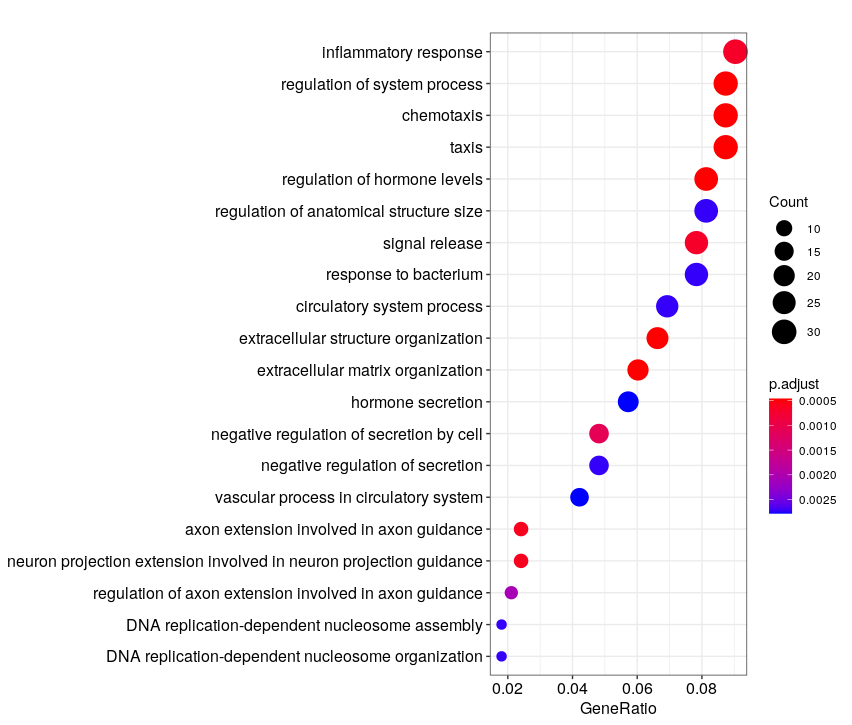``````# Reactome pathways enrichment
reactome.enrich <- enrichPathway(gene=as.character(unique(genes_DE\$ENTREZID)),
organism = "mouse",
qvalueCutoff = 0.01,
universe = genes_universe\$ENTREZID)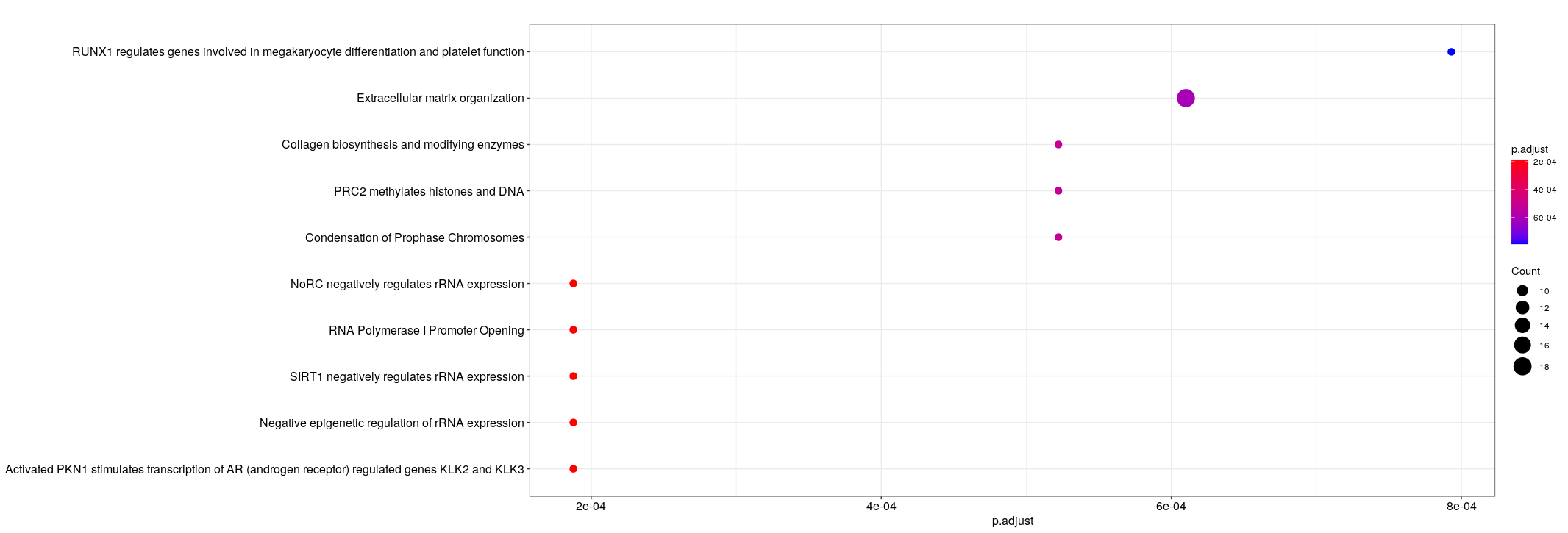## Additional : importing counts from salmon with `tximport`
The `tximport` R packages offers a fairly simple set of functions to get transcript-level expression quantification from salmon or kallisto into a differential gene expression analysis.
• If you have not computed them, you can find files with expression quantifications in : `/shared/data/Solutions/Liu2015/` and `/shared/data/Solutions/Ruhland2016/`